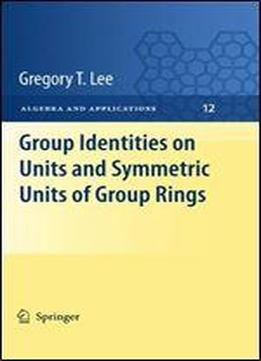# Group Identities On Units And Symmetric Units Of Group Rings (algebra And Applications) by Gregory T Lee / 2010 / English / PDF

Let FG be the group ring of a group G over a field F. Write U(FG) for the group of units of FG. It is an important problem to determine the conditions under which U(FG) satisfies a group identity. In the mid 1990s, a conjecture of Hartley was verified, namely, if U(FG) satisfies a group identity, and G is torsion, then FG satisfies a polynomial identity. Necessary and sufficient conditions for U(FG) to satisfy a group identity soon followed. Since the late 1990s, many papers have been devoted to the study of the symmetric units that is, those units u satisfying u* = u, where * is the involution on FG defined by sending each element of G to its inverse. The conditions under which these symmetric units satisfy a group identity have now been determined. This book presents these results for arbitrary group identities, as well as the conditions under which the unit group or the set of symmetric units satisfies several particular group identities of interest.

views: 223